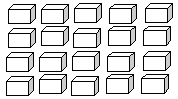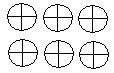Name: ___________________Date:___________________

 Email us to get an instant 20% discount on highly effective K-12 Math & English kwizNET Programs!

### Grade 2 - Mathematics7.9 Multiplication Review TestName: ___________________Date:___________________

### Grade 2 - Mathematics7.9 Multiplication Review Test

 Q 1: 5 x 5 =5201025 Q 2: Use the figure to find 5 times 4.211920 Q 3: Use the figure to find 3 times 2.467 Q 4: When two numbers are multiplied the answer is calleddifferencesumproduct Q 5: 4 x 7 =30282426 Q 6: 3 x 7 =none of the above41020 Q 7: 3 x 11 =none of the above223034 Q 8: Write the multiplication form for 4 + 4 + 4 + 44 X 4 = 164 + 4 = 84 X 3 = 12 Question 9: This question is available to subscribers only! Question 10: This question is available to subscribers only!

#### Subscription to kwizNET Learning System costs less than \$1 per month & offers the following benefits:

• Unrestricted access to grade appropriate lessons, quizzes, & printable worksheets
• Instant scoring of online quizzes
• Progress tracking and award certificates to keep your student motivated
• Unlimited practice with auto-generated 'WIZ MATH' quizzes